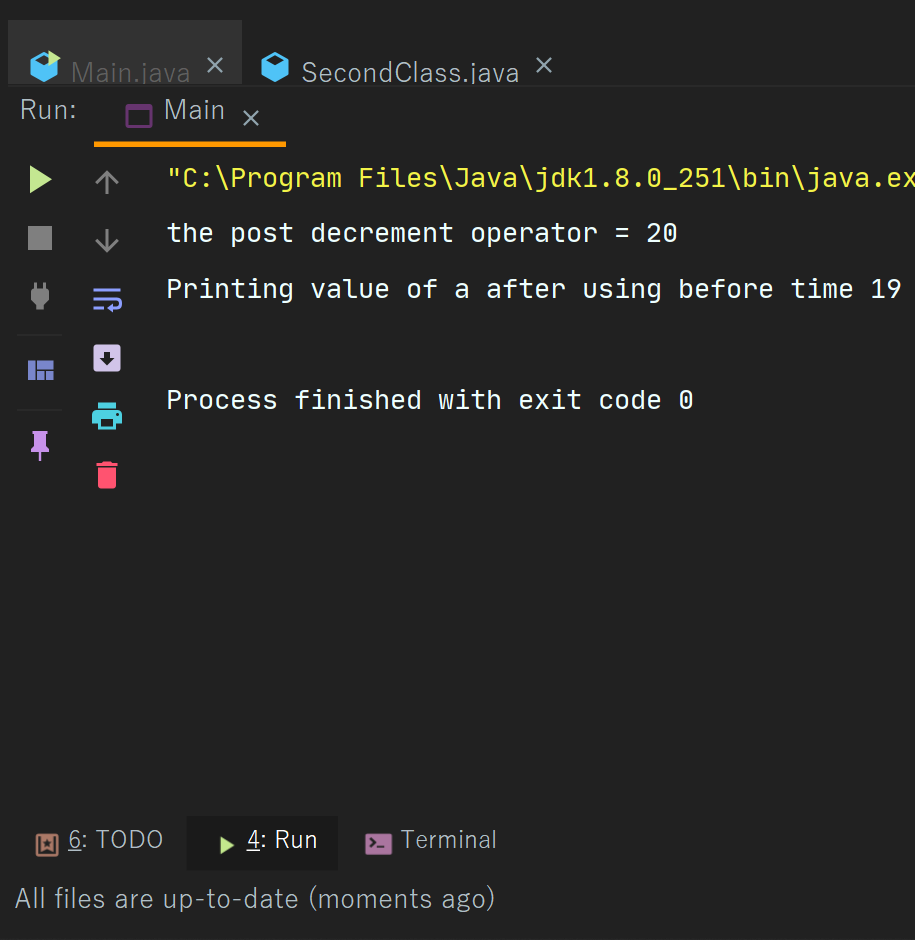Java Decrement Operators

# Java Decrement Operators

Decrement operator
If we want to subtract any value by one then we can use the unary operator which is of two types we will see both of the types as follows  -
Decrement operator is of two types-
Post increment- In post decrement --  comes after the value like a--, or num- - .
In this operator, the value is firstly used and then decrement.
Pre decrement - In this operator -- comes before the value like  --a, --b, and the value is first decreased and then used.
Now we will see examples -
Example -
 import second.*; import java.util.*;   // Pre decrement operator   class Main{   public static void main(String[] args) {       int a = 20;           System.out.println("the pre decrement operator = "+--a);             }   }
Output  -Example 2 -
 import second.*;   import java.util.*;   // Post decrement operator   class Main{   public static void main(String[] args) { int a = 20; System.out.println("the post decrement operator = "+a--);       System.out.println("Printing value of a after using before time "+a);       }   }
Output -